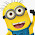## Tuesday, December 10, 2013

### InfoPath 2010: Calculate AVG and COUNT on multiple fields

A couple more calculations for InfoPath 2010:

To get the average of multiple Number fields, including a repeating table Number field, use the following:

Likewise, to get the total count of multiple fields that contain data, including a repeating table field, use the following:

More calculations will be posted as I deem necessary.

#### 6 comments:

1.Thanks a lot. This is what I was looking for!

2.How do I recreate the dividers between the fields? I haven't yet figured out an 'acceptable' way to do this in InfoPath.

Thanks

3.Pipes of course. Thanks

4.Buddy you are the gratest. THANKS!

5.how we can calculate the average of values like;
field 1 = 34:21:52 & field2 = 14:25:18

6.I am getting an error that the field I have the avg in, the final result is not an integer